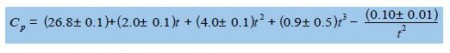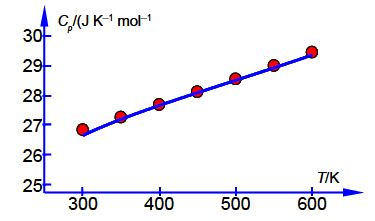# What is Heat Capacity of Solid lead?

The thermodynamic properties of solids and liquids are accurately modelled by the Shomate equation. For solids the Shomate equation is,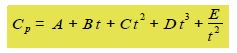Where Cp is in J K^-1 mol^-I and t = (T K)/1000. Below Figure shows the measured heat capacity Cp of solid Pb P P in column C from temperatures near room temperature at 300 K up to 600 K near its boiling point in column A, and in column B the temperatures t.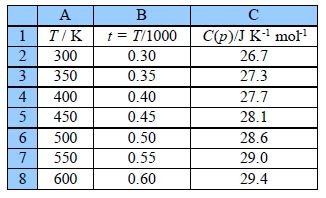Set up your own spreadsheet to solve the NLLSQ fitting for the Shomate equation for solid lead.

Solution: Heat Capacity of Solid lead

The solver spreadsheet for the Shomate equation is: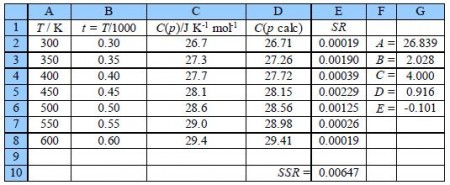Column D has the calculated heat capacity with D2 having,

= \$G\$2 + (\$G\$3*B2) + (\$G\$4*(B2^2)) + (\$G\$5*(B2^3)) + \$G\$6/(B2^2))

which refers to the temperature in kelvin/1000 and the location of the five arbitrary constants to A to E. Cell E2 takes the residual and squares it, i.e. the E2 is =(C2-D2)^2. The next question is the starting estimates for the constants, typing in unity into each of them gives SSR = 3037. Looking at the input data and the model equation then the heat capacities are in the 20s, so make A = 20 and typing in some values for B we find an initial value for the first temperature term B = 5.

This gives SSR = 63.4 when the other arbitrary constants are unity, this is a good enough starting point for iterating to the global minimum.

With the cursor on the SSR click on Tools, Solver, and in the Solver window, minimize and select cells G2 to G6 to vary. Click on Options and make sure the solver engine is DEPS; edit the number of learning cycles (iterations) from 2,000 to 2,000,000 and increase the stagnation number from 70 to 70,000.

It took 15.7 seconds and 1454 iterations to find the fit with SSR = 0.00647 which is a good fit, see below Figure. Finding a measure of the precision of the constants is carried out by changing the digits (and then undoing the change) systematically through the each constant until we find a change of about an order of magnitude in the SSR. This gives us a final Shomate equation which the final figure shown is the last significant digit. For clarity I have not included units in the equation. Below Figure shows a graph of the experimental data with our fitted line for the above Shomate equation.